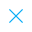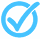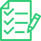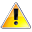Call Support +91-85588-96644

Keep me logged in
You can't leave Captcha Code empty
By submitting this form, you agree to the Terms & Privacy Policy.
ORTests givenPair of Linear Equations in Two Variables - 10

Questions

Time

Highest
score

Level

ENGLISH

Language

Topics Covered:Pair of Linear Equations in Two VariablesPair of Linear Equations in Two VariablesPair of Linear Equations in Two VariablesPair of Linear Equations in Two VariablesPair of Linear Equations in Two VariablesPair of Linear Equations in Two VariablesPair of Linear Equations in Two VariablesPair of Linear Equations in Two VariablesPair of Linear Equations in Two VariablesPair of Linear Equations in Two Variables

Latest TestsProbability - 3

30 Min - 15 Ques

Take TestPrime Number

30 Min - 20 Ques

Take TestBounds and Reciprocals

00 Min - 31 Ques

Take TestThis is a concept-building practice test and may not have exact structure as you would expect in the actual exam. Please exercise your discretion to attempt it or go to structured Featured Section.

Description:
Pair of Linear Equations in two Variables - 10